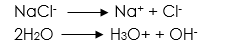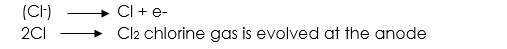Notice: Undefined index: options in /home/u337987201/domains/classnotes.ng/public_html/wp-content/plugins/elementor-pro/modules/theme-builder/widgets/site-logo.php on line 192
Electrolysis - ClassNotes.ng

# Electrolysis

Welcome to class!

In today’s class, we will be talking about electrolysis. Enjoy the class!

### ElectrolysisElectrolysis is the process by which the movement of an electric current through a solution liberates electrons. Chemicals in a container are decomposed to generate current.

Electrolytes are substances which either in their molten or soluble states allows the flow of an electric current with decomposition.

Thus, electrolysis is defined as the decomposition of compound or substance when in molten state or solutions by the passage of electric current.

#### Electrolyte

This is a substance or compound which conducts current, i.e. it contains mobile ions and undergoes decomposition, and is called an electrolyte, e.g. acids, bases, common salts.

#### Non-electrolyte

This is a substance which, either in molten state or in solution, does not allow the flow of an electric current.  Thus, it is a substance that does not conduct current or undergo decomposition.  Examples are organic solvents such as benzene, paraffin, sugar, salt, etc.

#### Electrodes

These are the two positive and negative pole dipped inside the electrolyte.  They are conductors because they allow the free flow of electrons through them. As a result, the current enters and leaves the electrolyte.

#### Anode

The anode is a positive [+Ve] electrode at which the electron leaves and current enters the electrolyte.

##### Cathode

This is a negative [-Ve] electrode at which the electron enters and current leaves the electrolyte.

#### Voltameter

The whole apparatus consisting of the vessel, electrolyte and electrodes is called the voltameter.

#### Ions

They are charged particles which exist in electrolytes and take part in electr0lysis.

These are the immediate products of decomposition of an electrolyte.  The ions which go to the anode are called anions; those ions which go to the cathode are called cations.

#### Electrical conduction through liquid and gases

Current electricity consists of fast-moving negative charge electrons. Current travels in materials which allow the flow of electrons called conductors. Current is produced in a simple circuit consisting of a battery [which is a source], a bulb [which is the lighting] and a tap key [which is used in controlling the simple system].

The current in the battery flows due to the force applied to it. The force is not visible but it is performed by some chemicals in the battery. The electrical pressure is called voltage.The Voltameter

Preferential Discharge of Ions; positively charged ions of the solute and the solvent move to the anode. Some ions are discharged in preference to others at the cathode and also at the anode.

Electrolysis of sodium chloride solution using carbon electrodes aqueous solution of sodium chlorideReaction

At the anode [using carbon]

(OH) ion and (Cl) ions moved to the anode Cl ions have a higher concentration than (OH), is discharged in preference to (OH)Reaction

At the cathode (using carbon)

Na+ and H3O+ ions moved the cathode

H3O+ is discharged in preference to Na+ ions.

H3O+ + e      →       H3O

2H3O           →          2H2O + H2

Hydrogen gas is liberated at the cathode.

At the anode = Cl2

At the cathode = H2

2HCl (solution formed)

Electrolysis of an aqueous solution of copper II tetraoxosulphate (VI) using platinum reaction at the anode

S02-4 and 0Hmoved to the anode.

0H ions are discharged in preference to the

S02-4 ion

0H          →               0H + e

40H         →               2H20 + 02

Oxygen gas is evolved at the anode. The reaction at the cathode

Cu2+ and H30+ ions would move to the cathode

Cu2+ ions are discharged at the cathode.

Cu2+ + 2e        →                Cu

Copper is deposited as the metal.

At the anode = 02

At the cathode = 2Cu

CuO (solution formed)

Michael Faraday stated two laws of electrolysis

Law 1: this law states that the mass of a substance liberated during electrolysis is directly proportional to the quantity of charge that passed through it.

Mathematically,

Mass of substance quantity of charge

Let the mass of a substance be represented as M

The quantity of charge be represented as Q

Since Q = it

Where I is current and t is the time taken

M = ZQ where Z is It

M = Zit

Z =  m/It

Where Z is a constant called electrochemical equivalent

Law 2: States that when the same quantity of electricity is passed through a solution of different electrolysis, the number of moles of the element deposited is inversely proportional to the charged on the ions at the elements.

Mathematically,

Number of moles depositedLet the number of moles deposited = m

Charge on the m = Q = it

According to the statementWorked example

(1) Find the mass of copper deposited on the cathode of copper voltameter if a current of 0.53A is passed through it for 30 minutes (e.c.e of copper = 3.3 x 10-4gC-1)

Solution

M = zIt

M = 3.3. x 10-4 x 0.53 x (30 x 60)

M = 0.315g

(2) The electrochemical equivalent of silver is 0.0012g/c. if 0.36g of silver is to be deposited by electrolysis on a surface by passing a steady current for 5minutes. Calculate the value of the current.

Solution

Given parameters

Z= 0.0012g/c

m = 0.36g

t = 5 minutes (5 x 60 = 300sec)

Therefore by applying Faraday’s first law of electrolysis

M = ZIt

0.36 = 0.0012 x I x 300

0.36 = 0.36I

I = 0.36/0.36

I = 1A

(3) In an electrochemical experiment, a cathode of mass 5g found to weigh 5.01g, after a current of 5A flows for 50Seconds. What is the electrochemical equivalent for the deposited substance?

Solution

Given parameters

M = 5.01 – 5 = 0.01g

t = 50 sec

I = 5A

M = ZIt

0.01 = Z x 5 x 50

0.01 = 250Z

Z= 0.01/250

Z = 4 x 10-5g/c

(4) A cooper and a silver voltameter are connected in series, and at the end of a period of time, 5.0g of copper was deposited, calculate the mass of silver deposited at the same time. Chemical equivalent of copper = 31.5. the chemical equivalent of silver = 108.Mass of silver deposited = 108 * 5/31.5

Mass of silver deposited = 17.14g

Presentation

1. Introduce the lesson by giving them definitions of Electrolysis.
2. Explain all the definitions by giving examples on each of them.
3. Pick out the keywords in the definitions and explain to them.
4. Ask them to State Faraday’s laws of electrolysis by using their own words and correct them if necessary.
5. Define electrode.
6. Solve simple calculation.
###### General evaluation
1. Name two industrial applications of electrolysis.

Assignment

(1) Explain the meaning of the following terms:

• Electrolysis
• Voltameter
• Electrode
• Electrolyte
• Cathode
• Anode

(2) How long will it takes for 1.5A current to coat evenly a layer of copper o.1mm thick on both sides of a 12 cm x 12 cm copper plate (e.c.e. of copper = 0.000331g/c; density of copper = 9g/cm3)

In our next class, we will be talking about Electric Measurement. We hope you enjoyed the class.

Should you have any further question, feel free to ask in the comment section below and trust us to respond as soon as possible.

For more class notes, videos, homework help etc download our Mobile App HERE

Join ClassNotes.ng Telegram Community HERE for exclusive content and support

Don`t copy text!